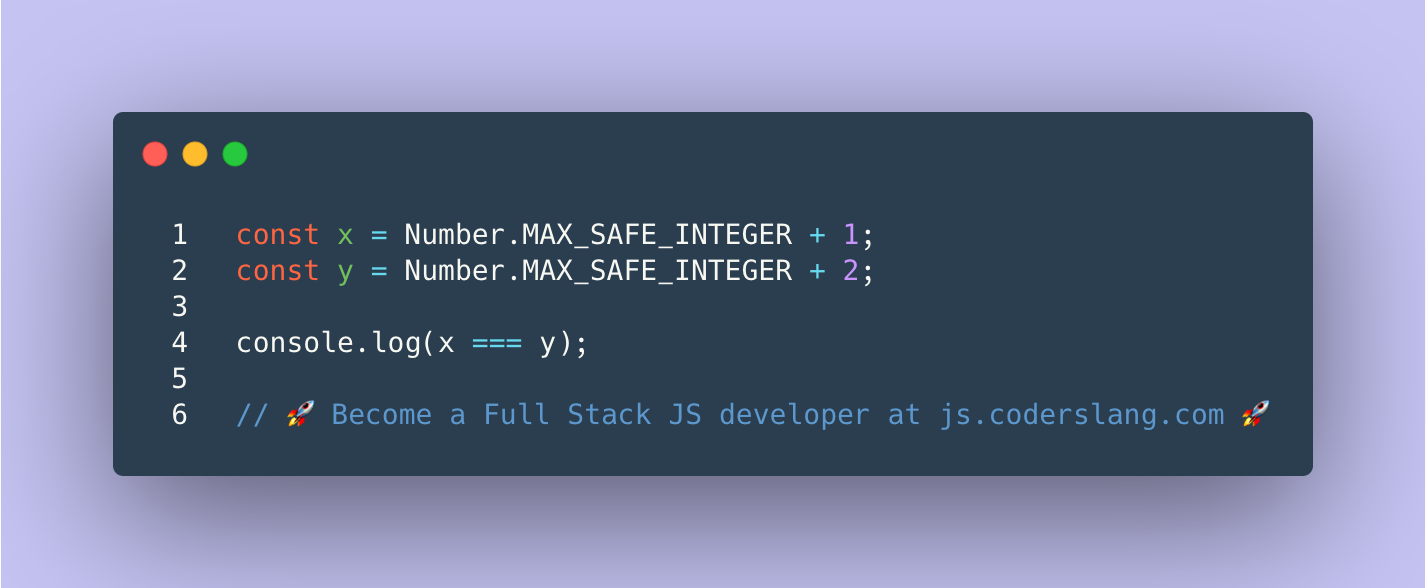True or false? What appears in the console?.

.

.

.

.

.

.

.

.

.

.

.

.

.

.

.

.

JavaScript uses the double precision floating point numbers even to represent integers. This means that the biggest number that can be stored safely as a JS number is 2^53^ - 1 or 9007199254740991. This value is stored as a static constant Math.MAX_SAFE_INTEGER.

console.log(Math.MAX_SAFE_INTEGER);      // 9007199254740991
console.log(Math.pow(2, 53) - 1);        // 9007199254740991

Having the value Math.MAX_SAFE_INTEGER doesn’t mean that it’s impossible to have a bigger number in JS. But, when we continue to increase the number, the loss of precision occurs.

console.log(Number.MAX_SAFE_INTEGER + 1); // 9007199254740992
console.log(Number.MAX_SAFE_INTEGER + 2); // 9007199254740992

As you see, by adding 1 and then 2 to Number.MAX_SAFE_INTEGER, we got the same number.

If you’re building the application where such behavior is critical, then you should use BigInt instead of the regular JavaScript Number.

ANSWER: There will be a loss of precision due to rounding and safe integer overflow. Both x and y will equal 9007199254740992. The message true will be printed to the console.